# Answers As of Present（Nirvana Spell）

## Answer to Aine & Binato's Puzzles

### Aine: Answer to 1st Puzzle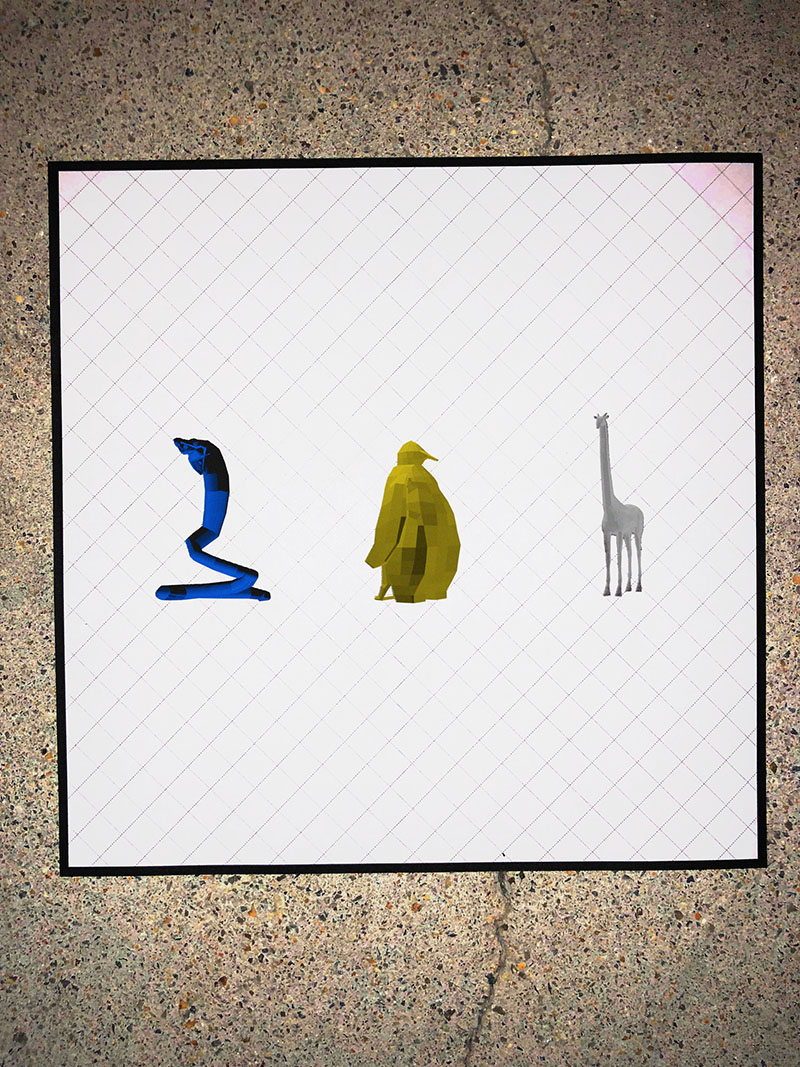#### ▼ Explanation

Observe corresponding animals in the video "Bats489."
"Blue Snake" = P
"Yellow Penguin" = A
"White Giraffe" = N

PAN

### Binato: Answer to 1st Puzzle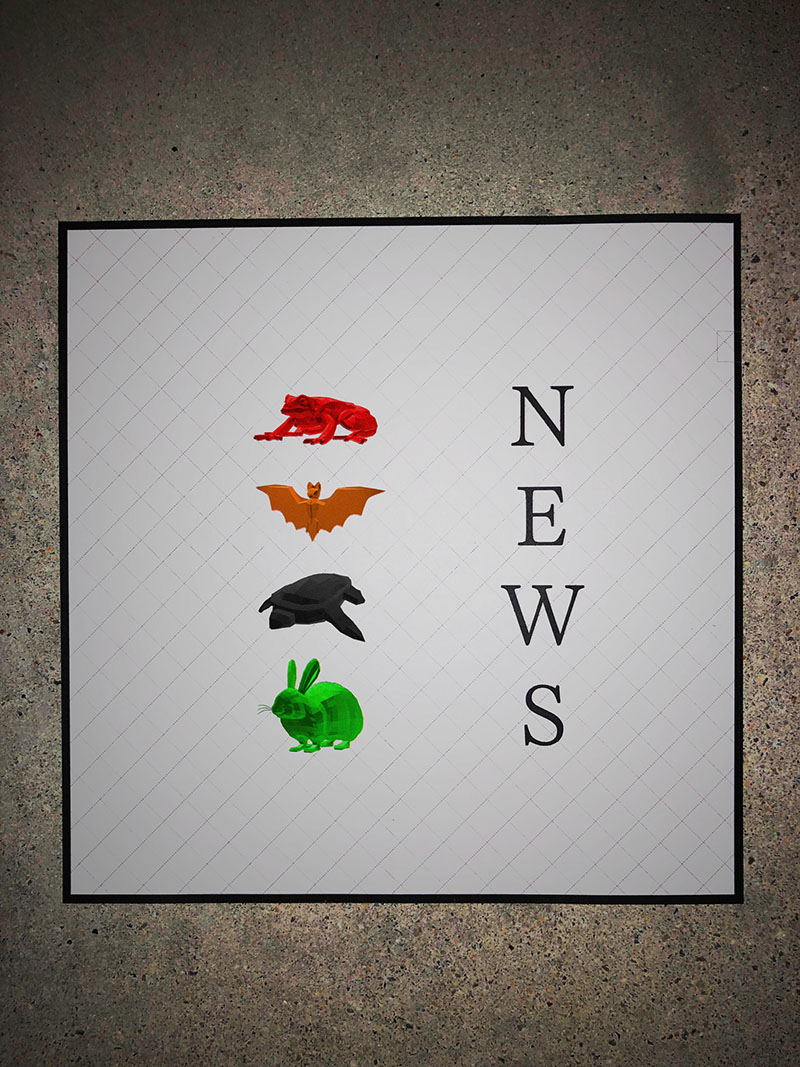#### ▼ Explanation

Observe corresponding animals in the video "Bats489."
"Red Frog" = 4 = 4th letter of NORTH = T
"Orange Bat" = 1 = 1st letter of EAST = E
"Black Tortoise" = 3 = 3rd letter of WEST = S
"Green Rabbit" = 4 = 4th letter of SOUTH = T

TEST

### Aine: Answer to 2nd Puzzle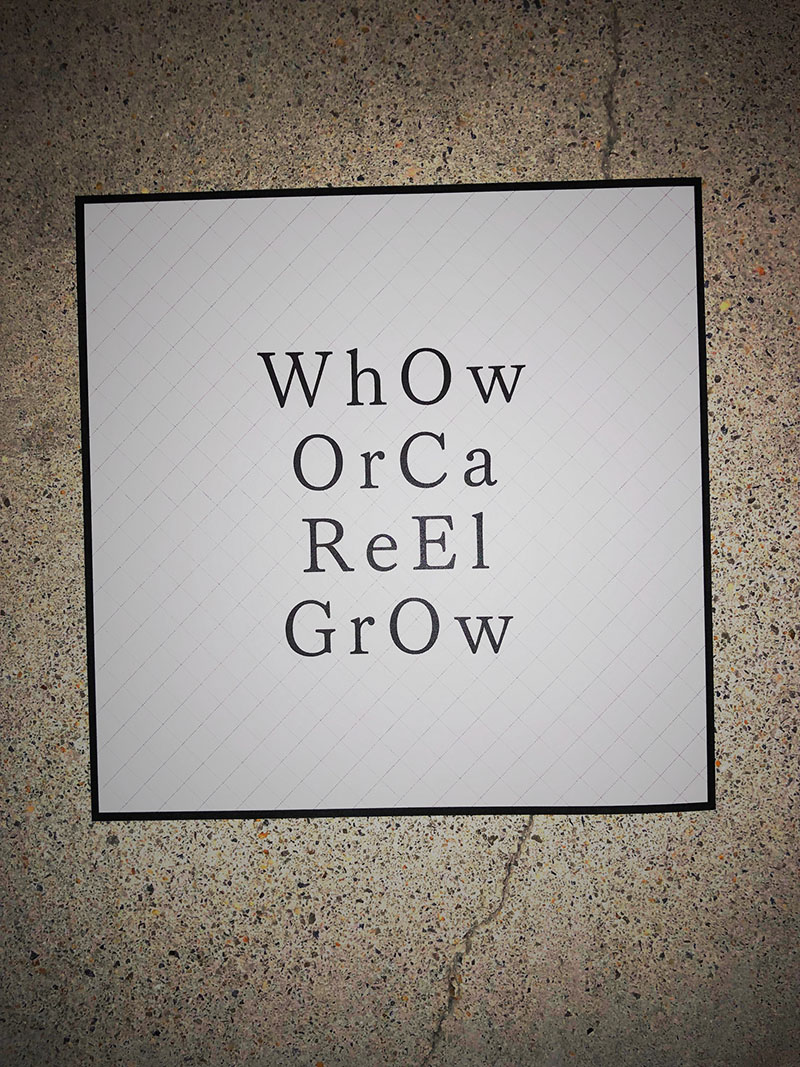#### ▼ Explanation

The first two English letters of the color + the first two English letters of the animal.
WhOw = White Owl = "White Owl" = C
OrCa = Orange Cat = "Orange Cat" = A
ReEl = Red Elephant = "Red Elephant" = K
GrOw = Green Owl = "Green Owl" = E

CAKE

### Binato: Answer to 2nd Puzzle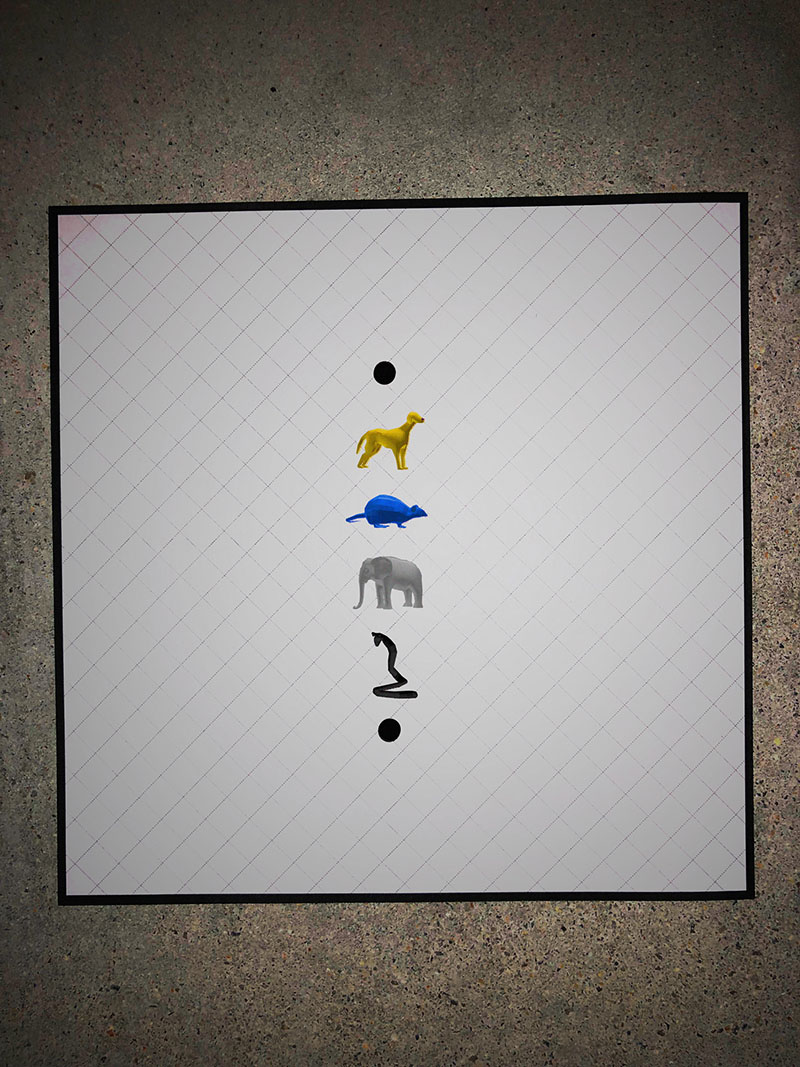#### ▼ Explanation

Corresponding to Morse code:
・ = E
"Yellow Dog" = ・・・ = S
"Blue Mouse" = －・－・ = C
"White Elephant" = ・－ = A
"Black Snake" = ・－－・ = P
・ = E

ESCAPE

### Aine: Answer to 3rd Puzzle#### ▼ Explanation

Observe where the Red -> Blue -> Yellow -> Blue -> Red pattern is found in the video "Bats489."
"Red Mouse" = S
"Blue Rabbit" = H
"Yellow Cat" = O
"Blue Dog" = E
"Red Rabbit" = S

SHOES

### Binato: Answer to 3rd Puzzle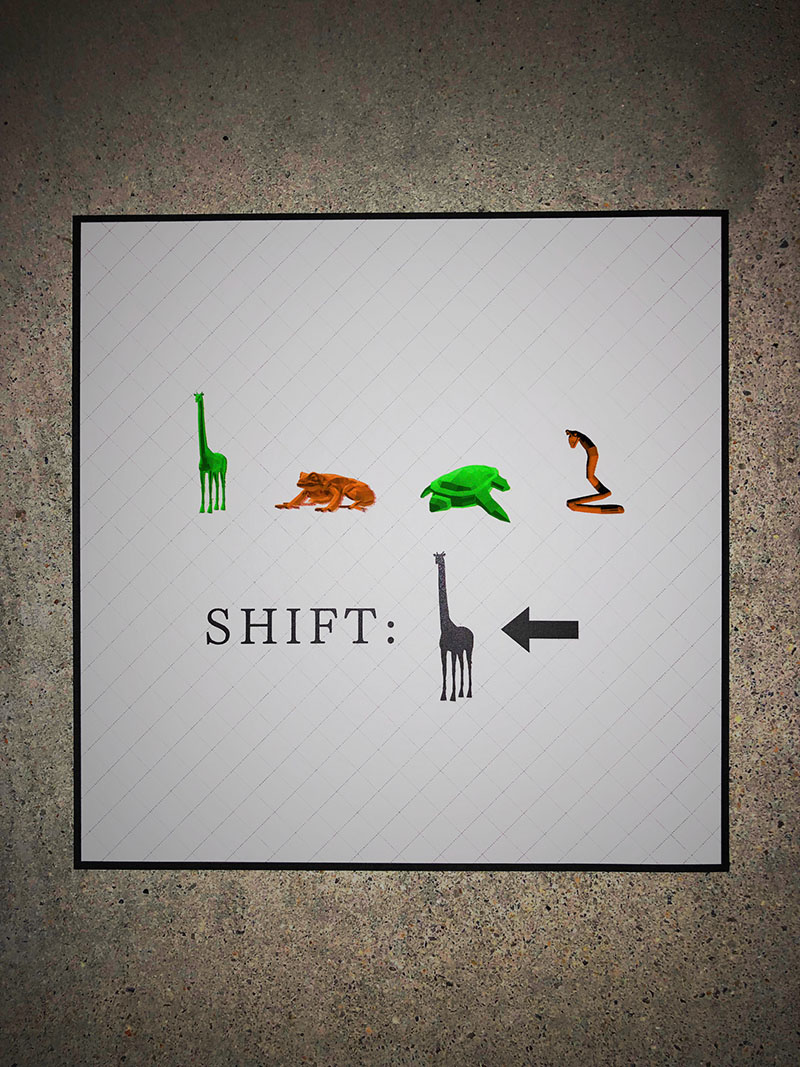#### ▼ Explanation

"Green Giraffe" = L
"Orange Frog" = F
"Green Turtle" = R
"Orange Snake" = J
"Black Giraffe" = 5
Decrypt using the "Caesar cipher" (5 shifts = shift 5 characters back)
L 5-character shift = G
F 5-character shift = A
R 5-character shift = M
J 5-character shift = E

GAME

## Answer to Mariha & Lumina's Puzzles

### Mariha: Answer to 1st Puzzle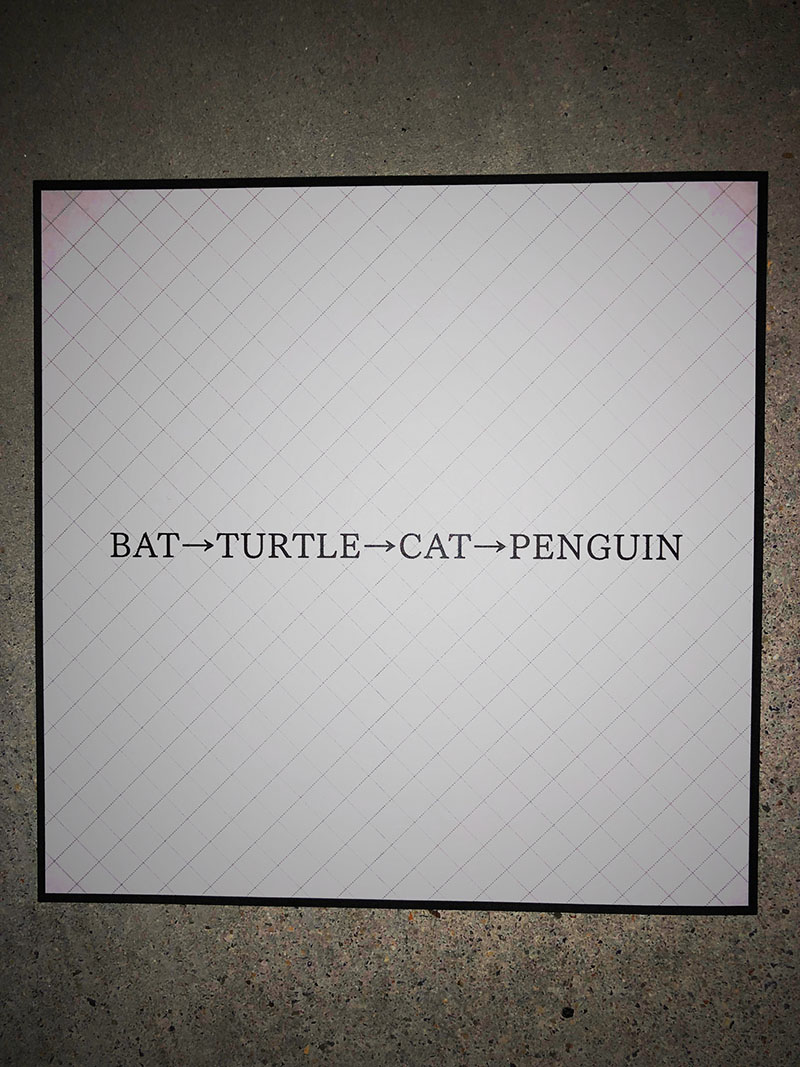#### ▼ Explanation

Observe the order of Bat -> Turtle -> Cat -> Penguin in the video "Bats489."

"Yellow Bat" = P
"White Turtle" = A
"Blue Cat" = I
"Black Penguin" = R

PAIR

### Lumina: Answer to 1st Puzzle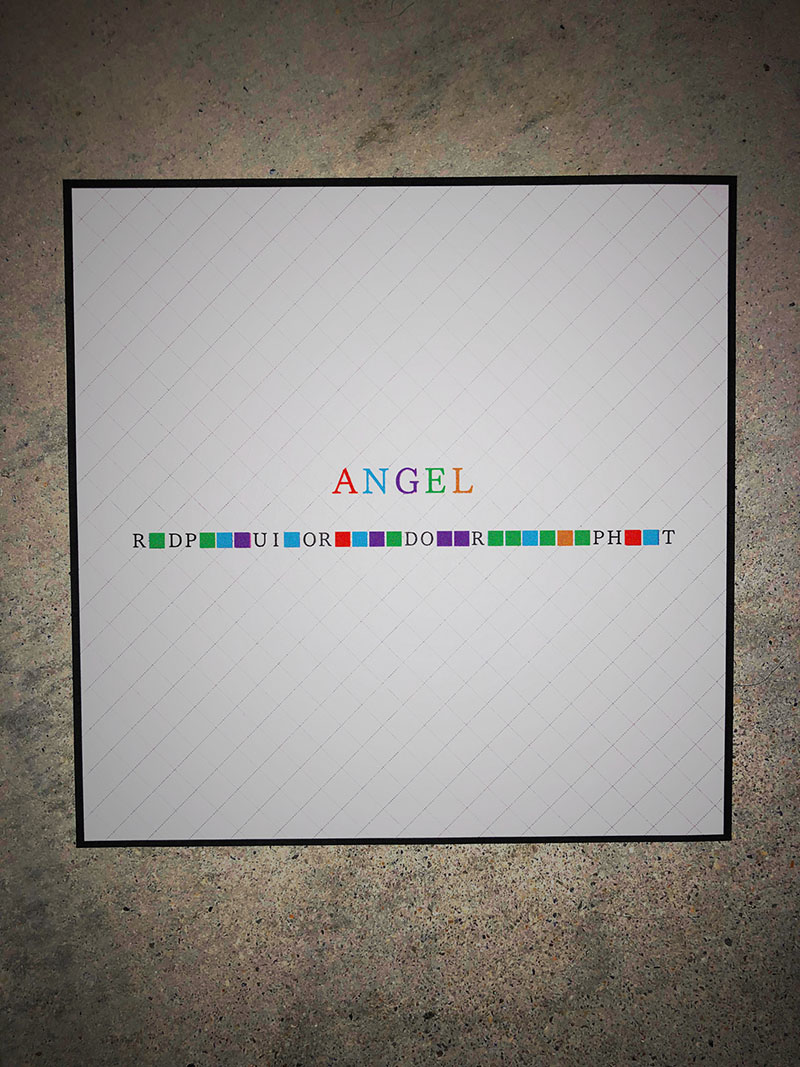#### ▼ Explanation

Red = A
Blue = N
Purple = G
Green = E
Orange = L
Substituting each character gives "RED PENGUIN ORANGE DOG GREEN ELEPHANT"
"Red Penguin" = S
"Orange Dog" = E
"Green Elephant" = T

SET

### Mariha: Answer to 2nd Puzzle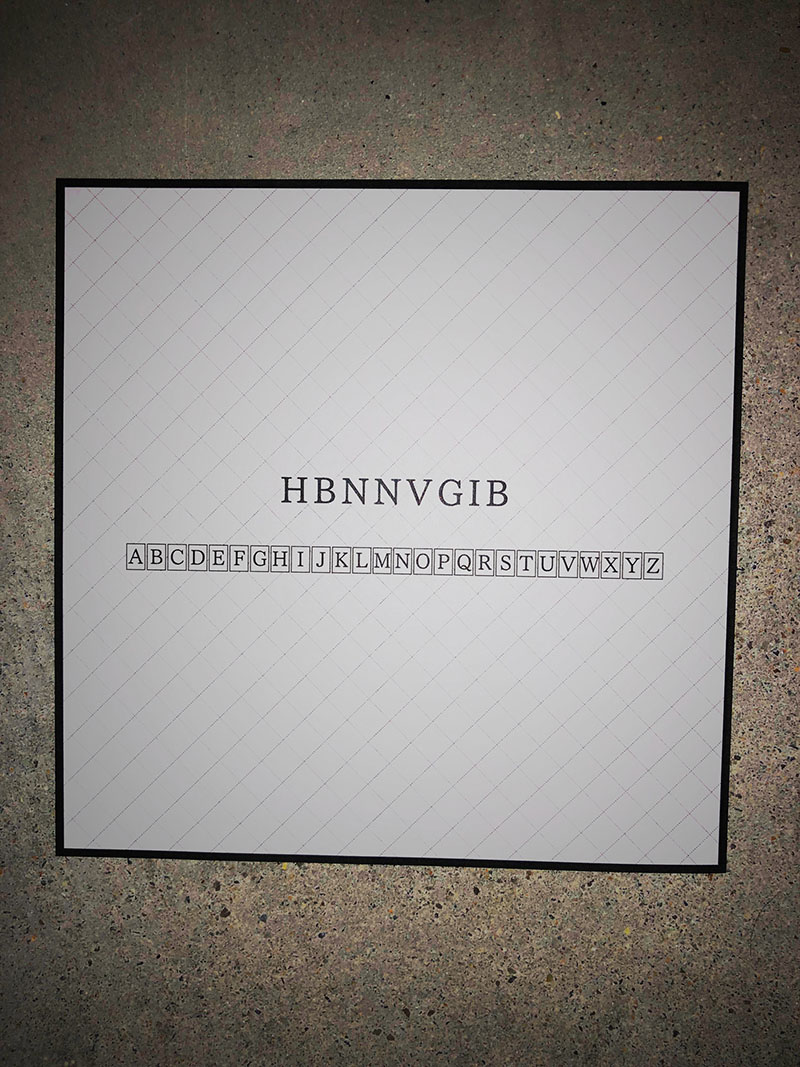#### ▼ Explanation

Observe the similar alphabet table in the video "Bats489."
Use the "Atbash cipher" for decryption.

The characters correspond as follows:
A = Z
B = Y
C = X
...
X = C
Y = B
Z = A

Decrypt "HBNNVGIB" according to this pattern.

SYMMETRY

### Lumina: Answer to 2nd Puzzle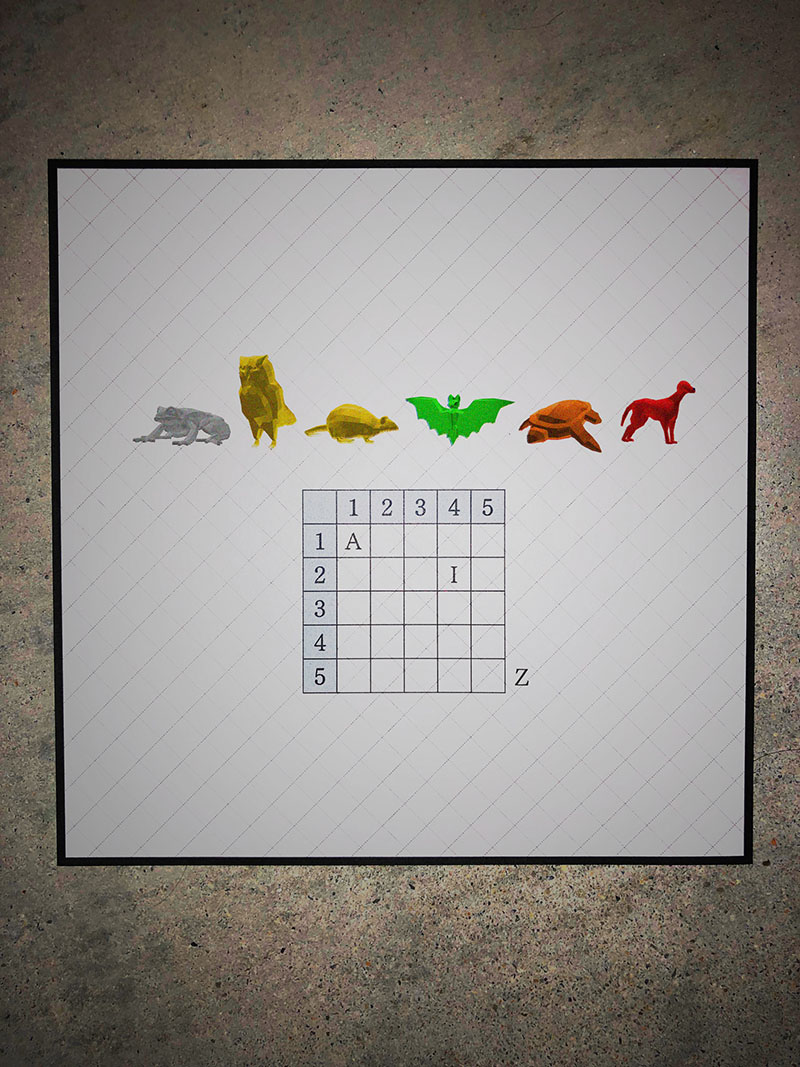#### ▼ Explanation

First, fill the table with the characters like a "Polybius Square."
Read the part corresponding to the vertical and horizontal numbers.
"White Frog" = 41 = D
"Yellow Owl" = 53 = O
"Yellow Mouse" = 15 = U
"Green Bat" = 21 = B
"Orange Turtle" = 23 = L
"Red Dog" = 51 = E

DOUBLE

### Mariha: Answer to 3rd Puzzle#### ▼ Explanation

Convert from Unicode to Emoji.
The emoji are in the video "Bats489."
・😀 (U+1F600) = M
・👁 (U+1F441) = U
・😢 (U+1F622) = T
・👁 (U+1F441) = U
・💀 (U+1F480) = A
・🙃 (U+1F643) = L

MUTUAL

### Lumina: Answer to 3rd Puzzle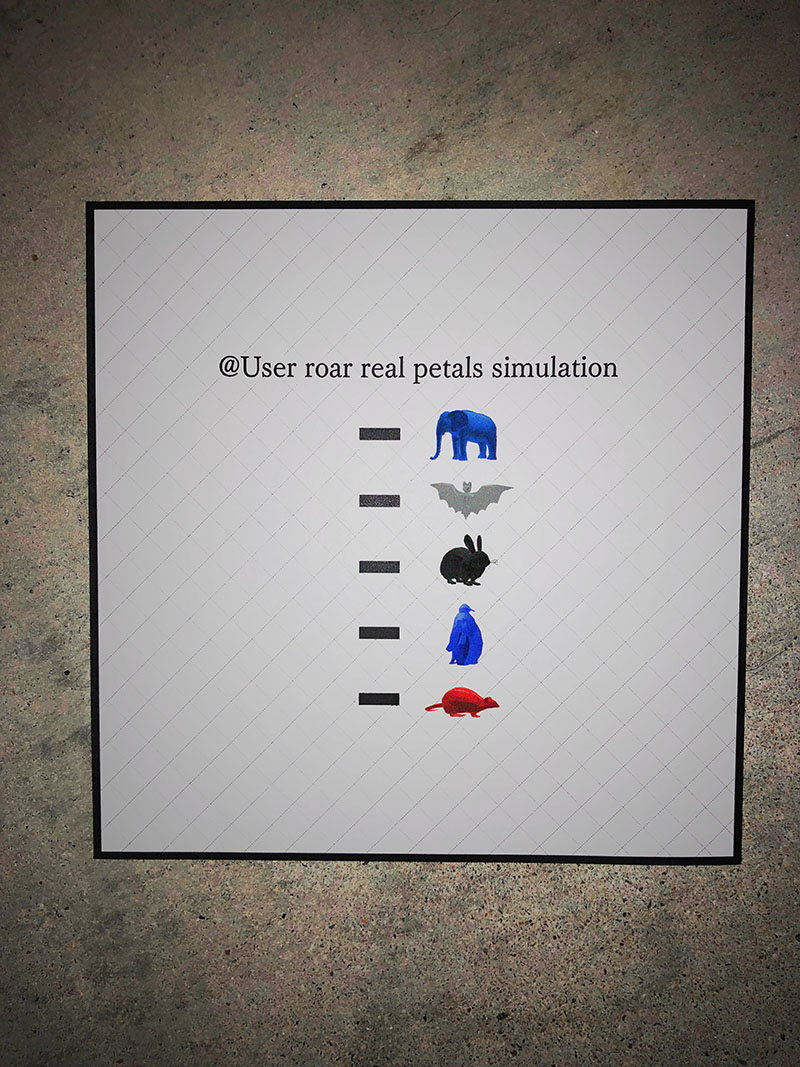#### ▼ Explanation

"Blue Elephant" = A
"White Bat" = L
"Black Rabbit" = M
"Blue Penguin" = U
"Red Mouse" = S

If you delete the above characters from "@User roar real petals simulation," it becomes "@ErrorRepetition."

Observe "@ErrorRepetition" tweet.

TWIN

### Mariha: Answer to 4th Puzzle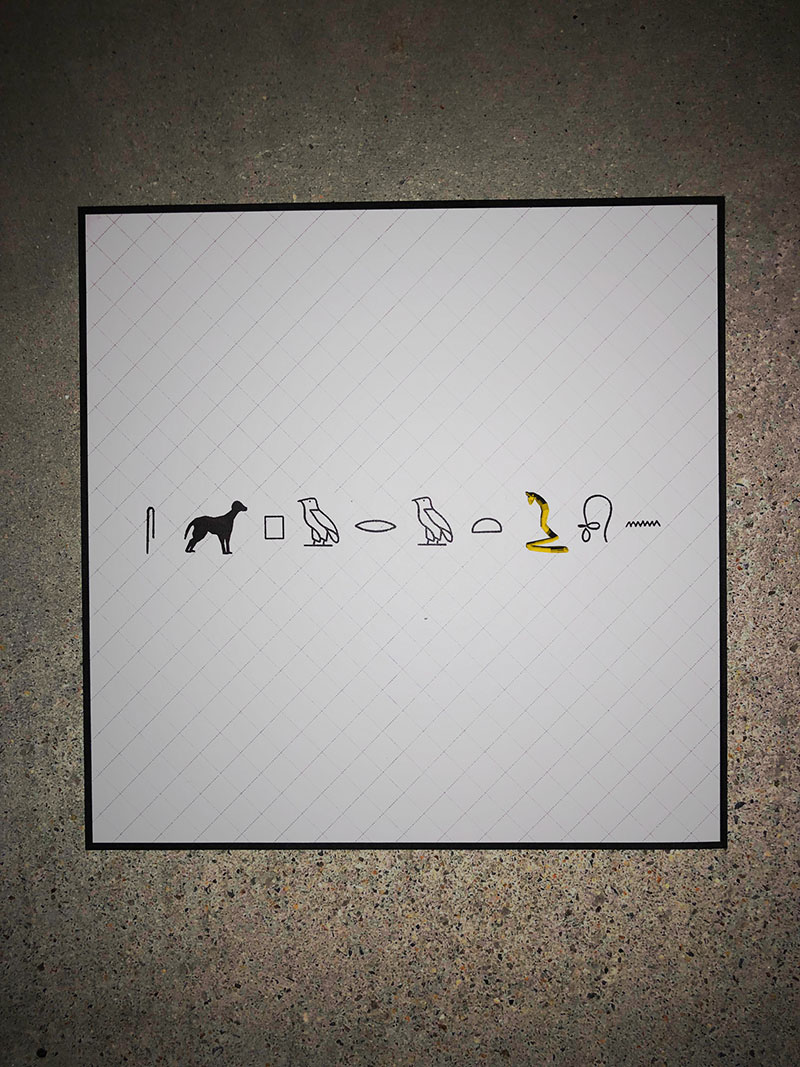#### ▼ Explanation

Read "Hieroglyphs" corresponding with the characters:
1st character = S
"Black Dog" = E
2nd character = P
3rd character = A
4th character = R
5th character = A
6th character = T
"Yellow Snake" = I
7th character = O
8th character = N

SEPARATION

### Lumina: Answer to 4th Puzzle#### ▼ Explanation

Use the "Pigpen Cipher" for the corresponding characters:
"Green Mouse" = C
1st symbol = O
2nd symbol = N
3rd symbol = F
4th symbol = L
5th symbol = I
"Green Mouse" = C
6th symbol = T

CONFLICT

## Answer to Iris & Kairo's Puzzles

### Iris: Answer to 1st Puzzle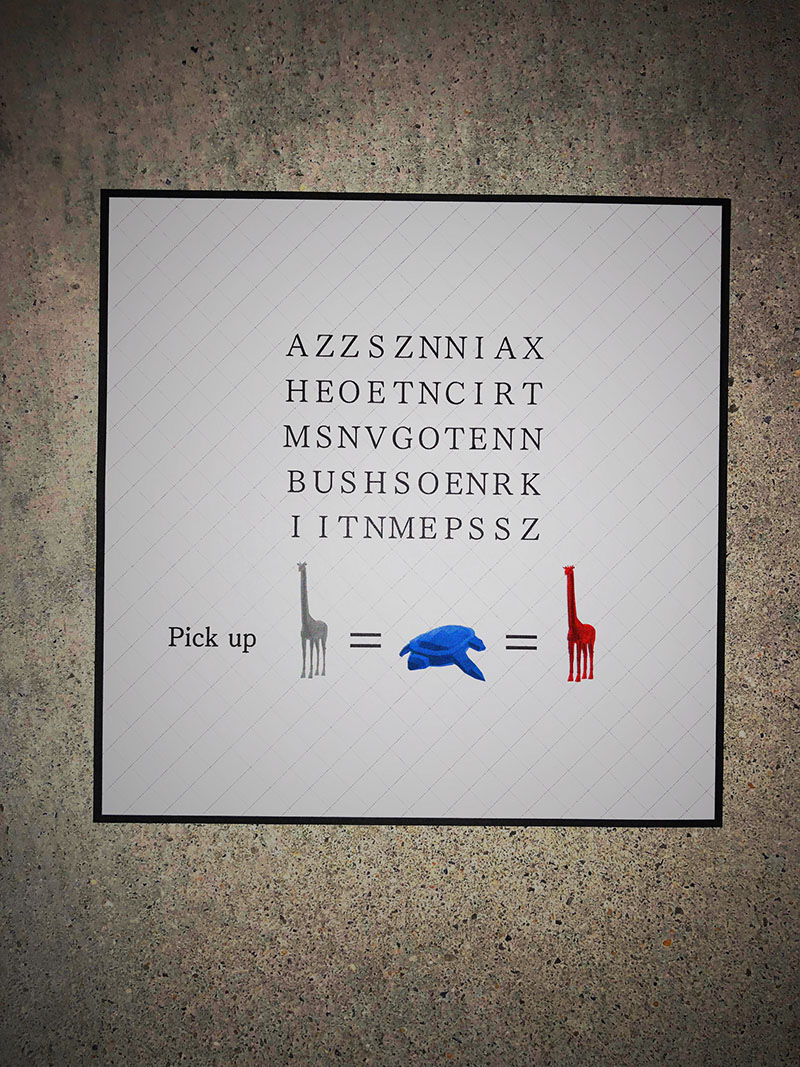#### ▼ Explanation

"White Giraffe" = N = nitrogen (element number 7)
"Blue Turtle" = ♅ = Uranus (7th planet)
"Red Giraffe" = 111 = 7 in binary
Because the common number is 7, this translates to "Pick Up: 7."
Pick every 7th character from the upper left of the character string.

NEMESIS

### Kairo: Answer to 1st Puzzle#### ▼ Explanation

"White Bat" = L
"Blue Cat" = I
"Black Rabbit" = M
"Black Penguin" = R
"Green Elephant" = T
"Yellow Bat" = P
The letters represent the English words for each part of a hand.

The numbers on each part of the hand in the image indicate a letter.
6: Little finger 6th character = E
2: Index finger 2nd character = N
2: Middle finger 2nd character = I
4: Ring finger 4th character = G
4: Thumb 4th character = M
2: Palm 2nd character = A

ENIGMA

### Iris: Answer to 2nd Puzzle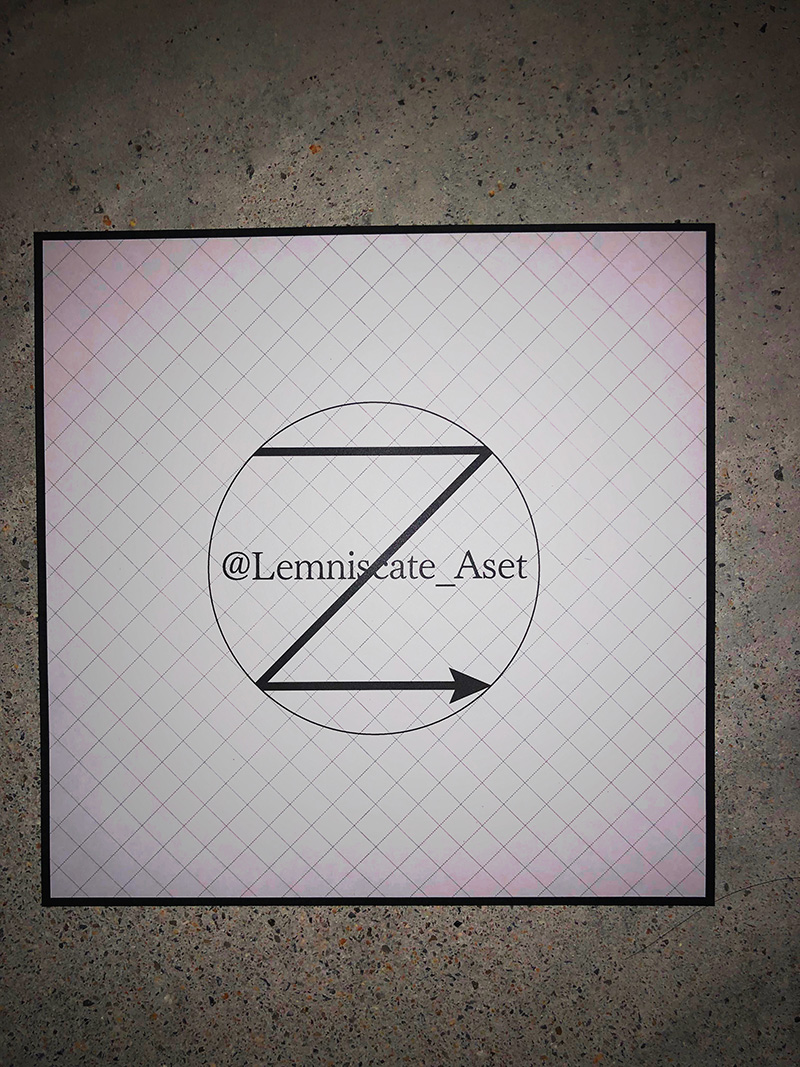#### ▼ Explanation

Animals are drawn in the four corners of Iris's Twitter account icon:
"Blue Rabbit" = H
"Blue Penguin" = U
"Green Turtle" = R
"Orange Owl" = T

*In the desktop version of Twitter, you can check the four corners of the icon by right-clicking the icon and opening the image in a new tab.

HURT

### Kairo: Answer to 2nd Puzzle#### ▼ Explanation

Find the Twitter account "@rm6b9lrw3szm."

Search for information on Twitter based on that account.

"White Cat" = T = Tweet = Tweet is the letter "C"
"White Penguin" = ♡ (heart) = Like = "A" for Liked tweets
"Blue Snake" = P = Profile = "U" in the profile
"Orange Frog" = F = Follow = The account name being followed "S"
"Yellow Rabbit & White Cat" = RT = "L" for Retweets
"Orange Giraffe" = H = Header = "I" in the header image
"Green Giraffe" = L = List = The list name created "T"
"Yellow Snake" = I = Icon = "Y" in the icon image

CAUSALITY

### Iris: Answer to 3rd Puzzle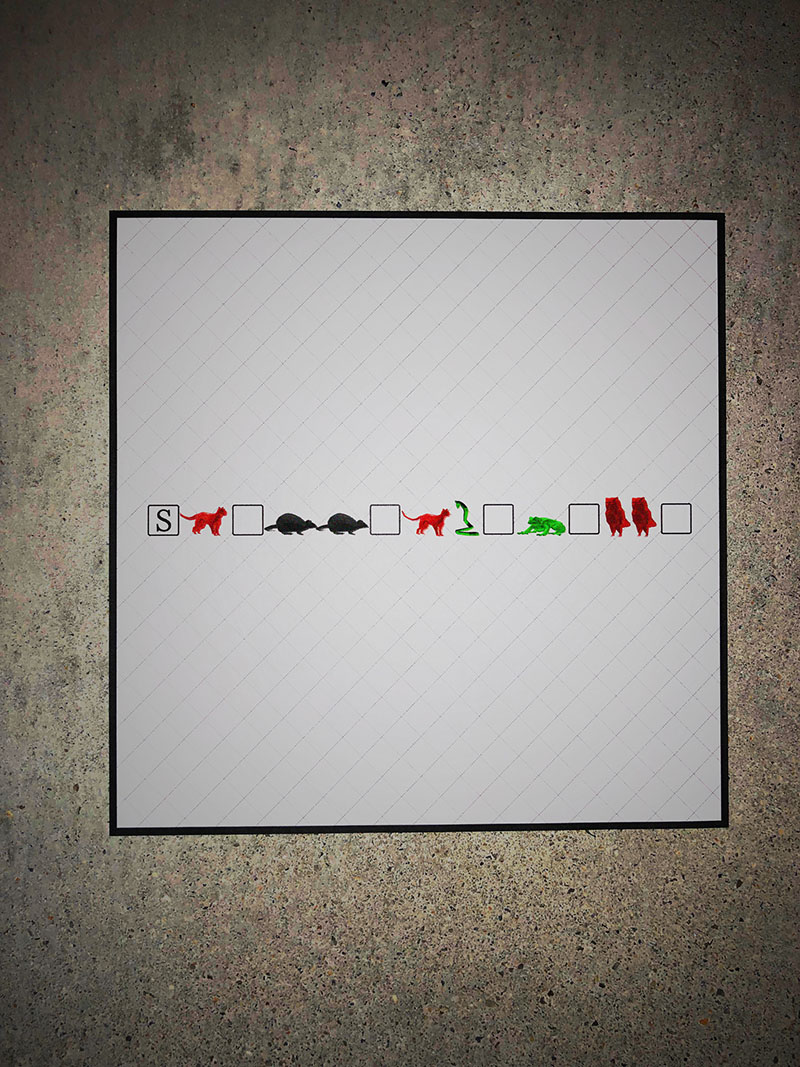#### ▼ Explanation

"Red Cat" = upper right arrow
"Black Mouse" = lower right arrow
"Green Snake" = upper left arrow
"Green Frog" = left arrow
"Red Owl" = right arrow
Starting with S on the keyboard, select the characters as indicated by the arrow.

SECRET

### Kairo: Answer to 3rd Puzzle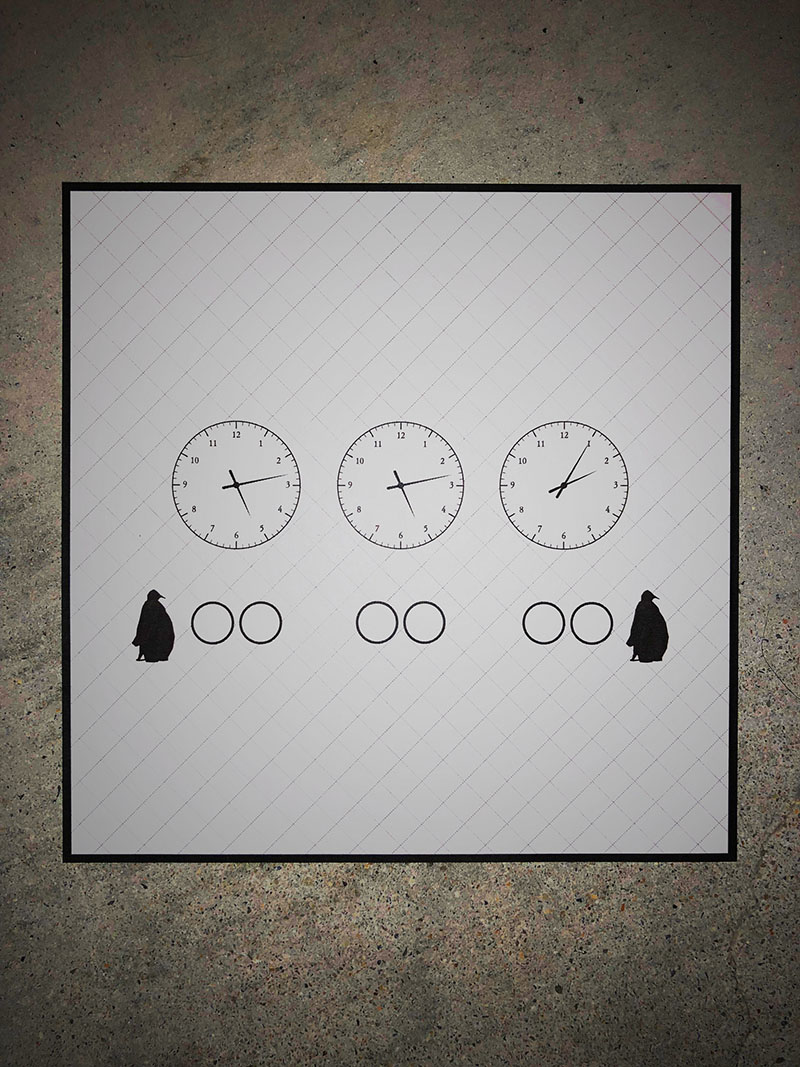#### ▼ Explanation

"Black Penguin" = R

The hands of the clock point to "5:13" "5:13" "2:05."
This indicates the letters:

5 = E
13 = M
5 = E
13 = M
2 = B
5 = E

Read by connecting these together.

REMEMBER

### Iris: Answer to 4th Puzzle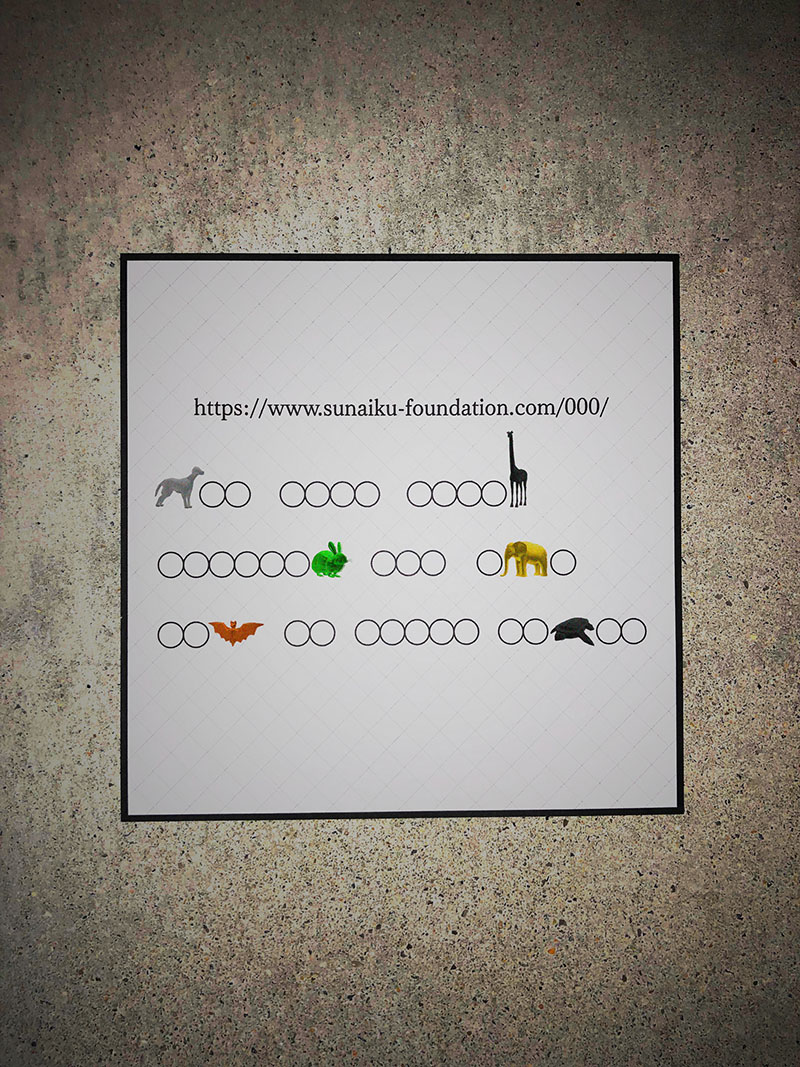#### ▼ Explanation

Looking at the URL of the image, the NOT FOUND page opens and the following message is displayed:
"The page you're looking for can not be found. Sorry."

Pick up the alphabet at the position of the animal in the image:
Position of T: "White Dog" = 6
Position of E: "Black Giraffe" = 5
Position of G: "Green Rabbit" = 4
Position of A: "Yellow Elephant" = 2
Position of T: "Orange Bat" = 1
Position of R: "Black Turtle" = 3

Read the alphabet in this numerical order.

TARGET

### Kairo: Answer to 4th Puzzle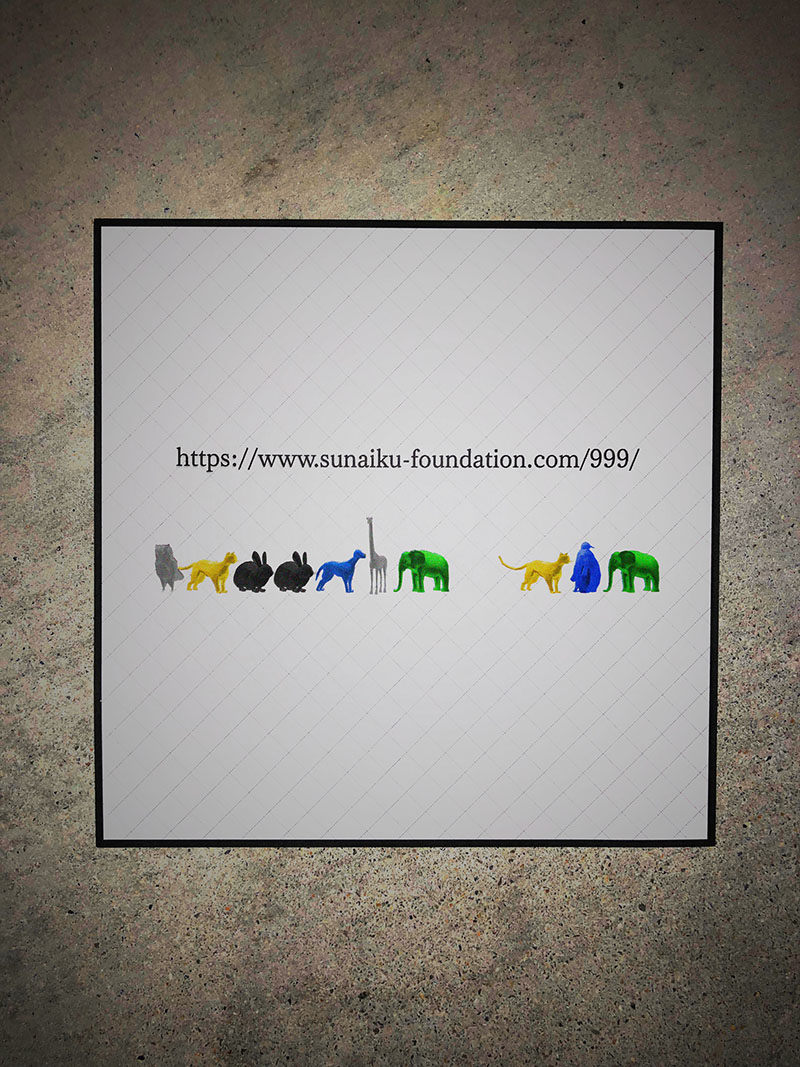#### ▼ Explanation

Open the URL of the image.

"White Owl" = C
"Yellow Cat" = O
"Black Rabbit" = M
"Blue Dog" = E
"White Giraffe" = N
"Green Elephant" = T
"Blue Penguin" = U
The letters spell "COMMENT OUT."

"COMMENT OUT" indicates that the source code is commented out.
Open the HTML of the URL.

The following commented out text is hidden in the HTML source:
<!-- Red Elephant, White Turtle, Yellow Rabbit, Black Cat, White Turtle -->

"Red Elephant" = K
"White Turtle" = A
"Yellow Rabbit" = R
"Black Cat" = M

KARMA

### Iris: Answer to 5th Puzzle#### ▼ Explanation

"Green Turtle" = R
"Orange Cat" = A
"Blue Cat" = I
"White Bat" = L
"Blue Giraffe" = F
"Orange Dog" = E
"Orange Elephant" = N
"Green Mouse" = C
"Black Turtle" = 3
The characters spell out "RAIL FENCE 3."

Using a "Rail Fence Cipher" (number of rails: 3) decoding "TSEEOTRNLTGSIRMPONAAIFJE" becomes "TRANSLATE GISEI FROM JP TO EN."

Translate Japanese "GISEI" into English.

SACRIFICE

### Kairo: Answer to 5th Puzzle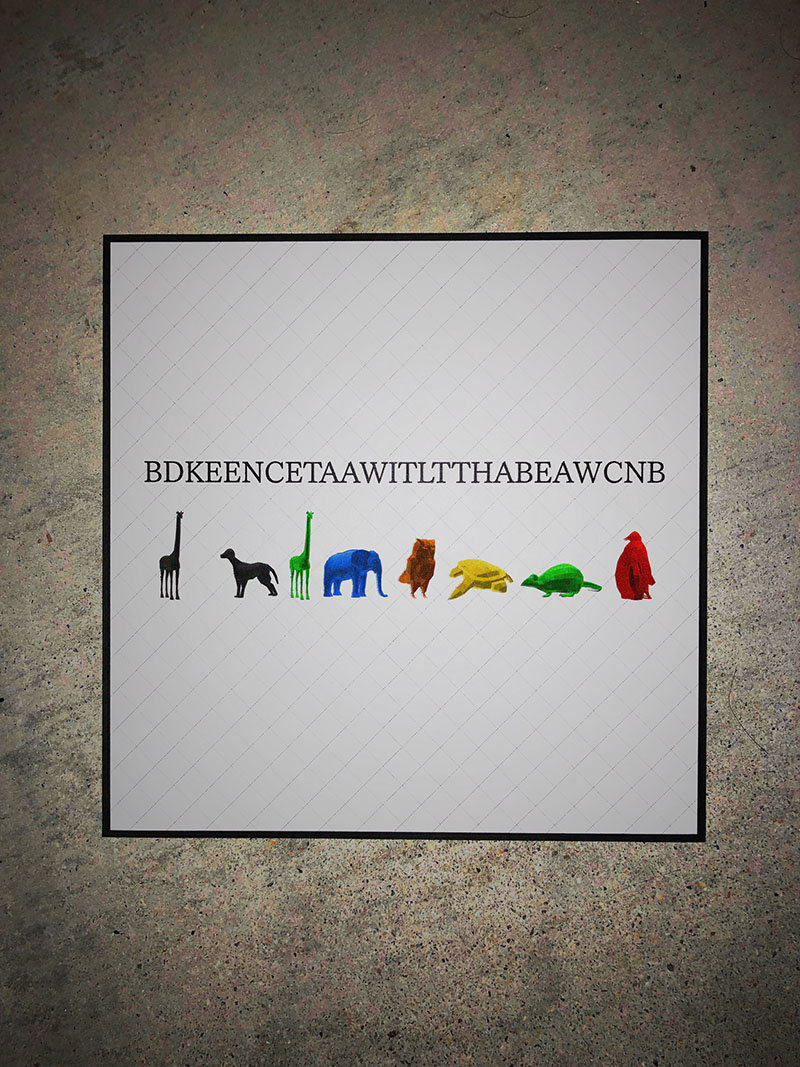#### ▼ Explanation

The picture of each animal is flipped horizontally, indicating that it is read in reverse.

"Black Giraffe" = 5
"Black Dog" = E
"Green Giraffe" = L
"Blue Elephant" = A
"Orange Owl" = T
"Yellow Turtle" = Y
"Green Mouse" = C
"Red Penguin" = S
It reads "SCYTALE 5" when read in reverse.

Use the "Scytale cipher" (pentagon).
In reverse, this becomes "BDKEENCETAAWITLTTHABEAWCNB" → "BNCWAEBAHTTLTIWAATECNEEKDB."

When decrypted, it becomes "BETWEEN BLACK CAT AND WHITE BAT."

Characters displayed between "Black Cat" and "White Bat" in the video "Bats489" = "ETAF."
Read "ETAF" in reverse.

FATE